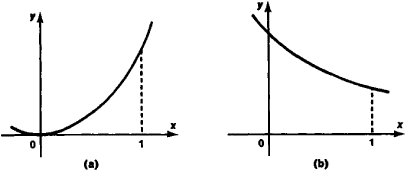# Increasing and Decreasing Functions

The following article is from The Great Soviet Encyclopedia (1979). It might be outdated or ideologically biased.

## Functions, Increasing and Decreasing

A function y = f(x) is called increasing over an interval [a, b] if for any pair of points x and*’, a ≤ x ≤ the inequality f(x)≤f(x’) is satisfied and strictly increasing if the inequality f(x)< f(x’) is satisfied. Decreasing and strictly decreasing functions are similarly defined. For example, the function y = x2 (Figure 1, a) strictly increases over the interval [0, 1], and y = l/(x 1) (Figure 1, b) strictly decreases over this interval. Increasing functions are designated as f(x)1 and decreasing functions a.sf(x) I. In order for a differentiable function f(x) to be increasing over an interval [a, b], it is necessary and sufficient that its derivative f’(x) be nonnegative over [a, b].Figure 1

In addition to the increase and decrease of a function over an interval, the increase and decrease of a function at a point are considered. The function y =f(x) is called increasing at a point Jt0 if an interval (α, β) can be found containing point x0 such that for any point x from (α, β), x > x0, the inequality f(x0) f(x) is satisfied and that for any point jc from (α, β), x ≤ x0, the inequality f(x) f(x) ≤ f(x0) is satisfied. The strict increase of a function at point x0 is similarly defined. If f(x0) > 0, then the function/fjcj strictly increases at point x0. lff(x) increases at every point of the interval (a, b), then it increases over this interval.

### REFERENCE

Fikhtengol’ts, G. M. Kurs differentsial’nogo i integral’nogo ischisleniia, 6th ed., vol. 1. Moscow, 1966.

S. B. STECHKIN

The Great Soviet Encyclopedia, 3rd Edition (1970-1979). © 2010 The Gale Group, Inc. All rights reserved.
Site: Follow: Share:
Open / Close